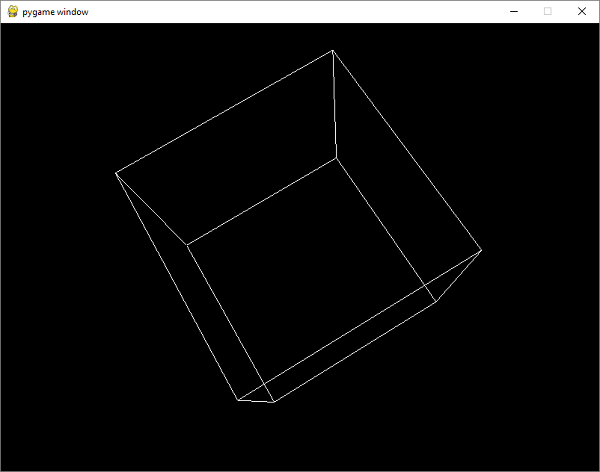# Pygame - PyOpenGL

OpenGL is a cross-language, cross-platform API for rendering 2D and 3D vector graphics. By interacting with a graphics processing unit (GPU), it achieves hardware-accelerated rendering. PyOpenGL library is Python’s binding for OpenGL.

We need to install it using pip utility −

pip3 install pyopengl


First we shall import functions from OpenGL.GL and OpenGL.GLU (utility functions) modules.

OpenGL specifies objects within the space by defining vertices or nodes. Lines between vertices are called edges. The OpenGL code is written between glBegin and glEnd.

In our example, we shall draw a cube with following vertices and edges −

verticies = (
(1, -1, -1),
(1, 1, -1),
(-1, 1, -1),
(-1, -1, -1),
(1, -1, 1),
(1, 1, 1),
(-1, -1, 1),
(-1, 1, 1)
)
edges = (
(0,1),
(0,3),
(0,4),
(2,1),
(2,3),
(2,7),
(6,3),
(6,4),
(6,7),
(5,1),
(5,4),
(5,7)
)


The cube() function performs OpenGL drawing −

def Cube():
glBegin(GL_LINES)
for edge in edges:
for vertex in edge:
glVertex3fv(verticies[vertex])
glEnd()


The GL_LINES attribute to glBegin() tells that lines are to be drawn.

We need to specify OPENGL and DOUBLEBUF flags in set_mode() function that sets up the display.

pygame.display.set_mode(display, DOUBLEBUF|OPENGL)


Then call the gluPerspective() determines the perspective. The first parameter is the degree of the field of view. The second value is the aspect ratio. The next two values here are the znear and zfar, which are the near and far clipping planes.

gluPerspective(45, (display/display), 0.1, 50.0)
glTranslatef(0.0,0.0, -5)


Inside the Pygame event loop, first rotate the current matrix, clear the color buffer and depth buffer, and call cube() function. Finally, we update the display window.

while True:
for event in pygame.event.get():
if event.type == pygame.QUIT:
pygame.quit()
quit()

glRotatef(1, 3, 1, 1)
glClear(GL_COLOR_BUFFER_BIT|GL_DEPTH_BUFFER_BIT)
Cube()
pygame.display.flip()
pygame.time.wait(10)


## Example

The complete code of the example is as follows −

import pygame

from pygame.locals import *

from OpenGL.GL import *
from OpenGL.GLU import *

verticies = (
(1, -1, -1),
(1, 1, -1),
(-1, 1, -1),
(-1, -1, -1),
(1, -1, 1),
(1, 1, 1),
(-1, -1, 1),
(-1, 1, 1)
)
edges = (
(0,1),
(0,3),
(0,4),
(2,1),
(2,3),
(2,7),
(6,3),
(6,4),
(6,7),
(5,1),
(5,4),
(5,7)
)
def Cube():
glBegin(GL_LINES)
for edge in edges:
for vertex in edge:
glVertex3fv(verticies[vertex])
glEnd()

def main():
pygame.init()
display = (800,600)
pygame.display.set_mode(display, DOUBLEBUF|OPENGL)

gluPerspective(45, (display/display), 0.1, 50.0)

glTranslatef(0.0,0.0, -5)

while True:
for event in pygame.event.get():
if event.type == pygame.QUIT:
pygame.quit()
quit()
glRotatef(1, 3, 1, 1)
glClear(GL_COLOR_BUFFER_BIT|GL_DEPTH_BUFFER_BIT)
Cube()
pygame.display.flip()
pygame.time.wait(10)

main()


## Output

Run the above code. You will see a rotating cube on Pygame’s window surface. This is a short demonstration of capability of PyOpenGL. A detailed discussion of this library is beyond the scope of this tutorial.